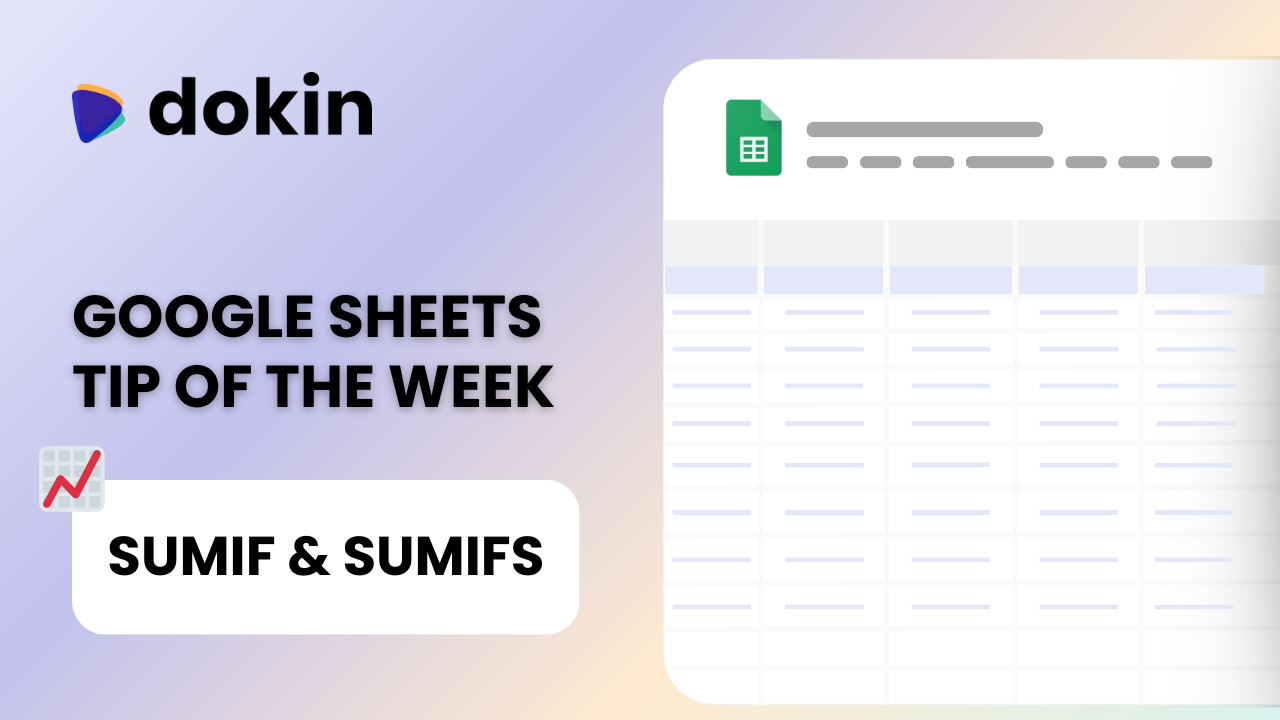# How to use SUMIF & SUMIFS functions in Google Sheets

/
October 21, 2023## Introduction

Looking to sum data from a range or array based on 1 or multiple conditions?

For the Google Sheets tip of this week we'll talk about SUMIF & SUMIFS. These 2 Google Sheets functions allow you to sum data from a range based on one or multiple conditions.## How do I use Sumif in Google Sheets?

SUMIF is a function in Google Sheets designed to simplify data analysis. It allows you to calculate the sum of a range of values based on specific criteria. For instance, you can use SUMIF to add up all the sales made by a particular salesperson or the total revenue generated from a specific product.

Syntax:

=SUMIF(range, criteria, [sum_range])

Here,

• range is the range of cells you want to apply the criteria to.
• criteria is the condition that the cells must meet to be included.
• [sum_range] is the range of cells to be summed. If omitted, the range is summed.

Using SUMIF is straightforward:

1. Select the cell where you want the result to appear.
2. Enter the SUMIF formula with the appropriate range, criteria, and optional sum_range.
3. Press Enter, and Google Sheets will calculate the sum based on your criteria.

## How do I use Sumifs with multiple criteria in Google Sheets?

If you need to apply multiple criteria to your summing, you can use the SUMIFS function.

SUMIFS allows you to sum values that meet multiple conditions. Particularly useful for example whe you have data sets with different column and want to sum a specific array with conditions from different columns

The syntax is as follows:

=SUMIFS(sum_range, criteria_range1, criteria1, criteria_range2, criteria2, ...)

As you can see, in this case the sum_range is the first string that is called, and then each criteria range, followed by the criterion itself.

Here's how to use SUMIFS:

1. Select the cell where you want the result.
2. Enter the SUMIFS formula with the sum_range and pairs of criteria_range and criteria.
3. Press Enter, and Google Sheets will calculate the sum based on multiple criteria.

## When do you use Sumifs function?

Sumifs is particularly useful when you have complex data filtering needs. For instance, you can sum sales for a specific product in a particular region within a certain timeframe. The flexibility of SUMIFS makes it a valuable tool in your data analysis toolkit.

### How do I sum if a cell contains specific text?

Sometimes, you want to sum values if a cell contains specific text. For this, you can use a combination of functions, including SUMIF or SUMIFS, with a wildcard character like *. Here's how you can do it:

Let's say you want to sum all sales where the product name contains the word "Widget." You can use the following formula:

=SUMIF(range, "Widget", [sum_range])

## Conclusion

SUMIF and SUMIFS in Google Sheets are valuable tools, especially useful when you need a quick solution to calculate a sum from items on a range.